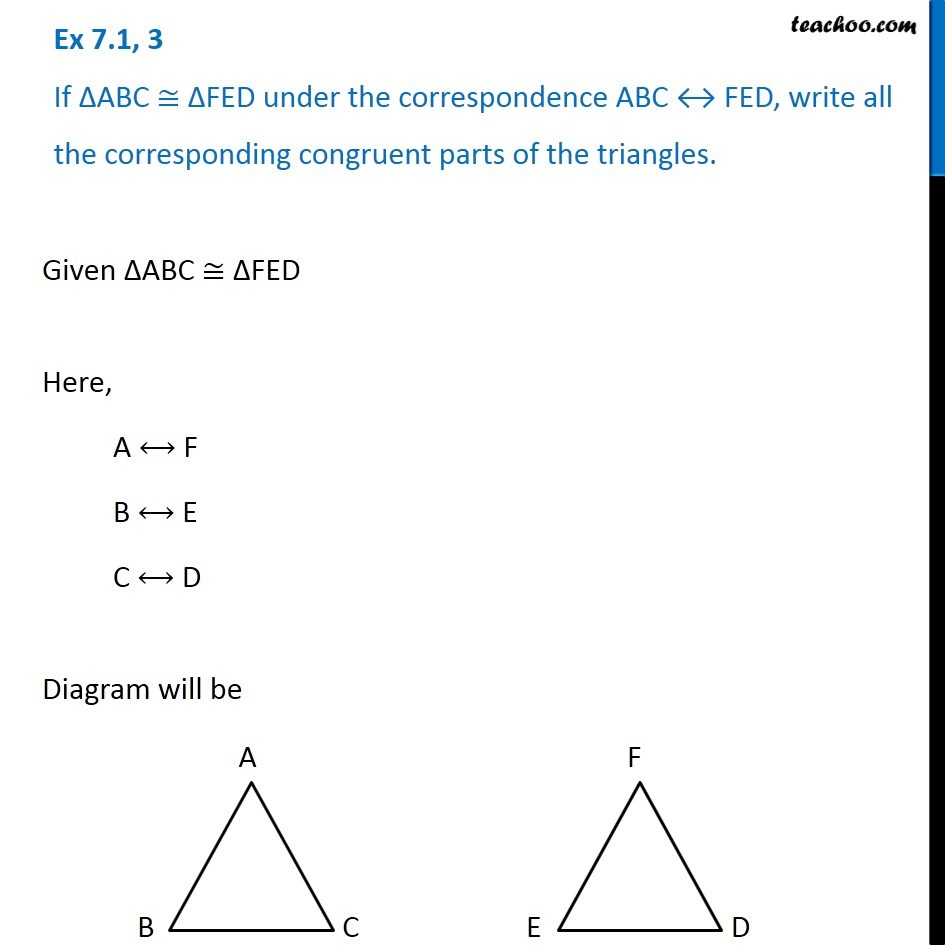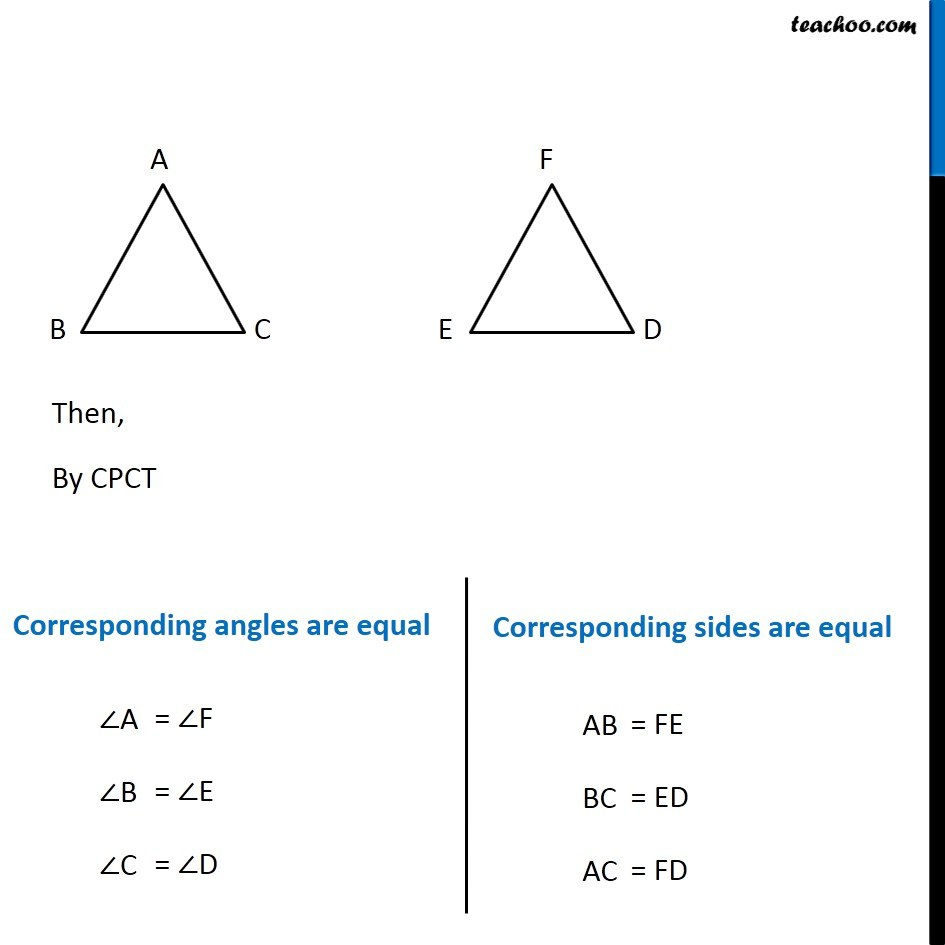1. Chapter 7 Class 7 Congruence of Triangles
2. Concept wise
3. Congruency of triangles

Transcript

Ex 7.1, 3 If ∆ABC ≅ ∆FED under the correspondence ABC ↔ FED, write all the corresponding congruent parts of the triangles. Given ∆ABC ≅ ∆FED Here, A ⟷ F B ⟷ E C ⟷ D Diagram will be Then, By CPCT Corresponding angles are equal ∠ A = ∠ F ∠ B = ∠ E ∠ C = ∠ D Corresponding sides are equal AB = FE BC = ED AC = FD